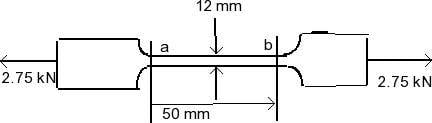# Stress and Strain Problem

• fnicolep92

## Homework Statement

A 2.75 KN tensile load is applied to at test coupon made from 1.6 mm flat steel plate (E=200 GPa, v=0.30). Determine the resulting change in the 50 mm gage length.## Homework Equations

εx = (σx/E) + (-Vσy/E) - (Vσz/E)
εy = (-vσx/E) + (σy/E) - (Vσz/E)
εz = (-vσx/E) - (Vσy/E) + (σz/E)

## The Attempt at a Solution

(a) Area = (0.05)(0.0016) = 0.00008 m2
σx = P/A = (2.75*103) / 0.00008
σx = 34.4*106 Pa

εx = (34.4*106) / (200*109)
εx = 1.719*10-4

Δab = εx(ab) = (1.719*10-4 / 0.05
Δab = 8.595*10-6

The answer should be 0.0358 mm. What am I doing wrong?

For one thing, your cross sectional area is 1.6x12, not 1.6x50. Also, you should be multiplying by 50 in getting Δab, not 0.05.

Chet